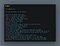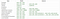# Learning The Pythonic Way

## Some cool features and tips to write more Pythonic codePhoto by Tudor Baciu on UnsplashThe Zen of Python

## Here are a few practices and features that will help you write idiomatic and Pythonic code:

• Naming Conventions: The PEP8 (Python Enhancement Proposal) guidelines suggest some specific naming conventions such as:Naming Conventions in Python
`# assigning a and b as 100a = b = 100`
`# Iterable UnpackingX Not recommendedemployees = ["Carlos", "Badal", "Manoj"]first = employeessecond = employeesthird = employees=> Recommendedfirst, second, third = employeesXX ValueError: too many values to unpack if : first, second, third = ["Carlos", "Badal", "Manoj", "Michael"] XX ValueError: not enough values to unpack if :first, second, third = ["Carlos", "Badal"]# Unpacking generatorsresult = (i ** 3 for i in range(5))zero, one, two, three, four = result# Unpacking a dictionarydicts = {'one': 1, 'two':2, 'three': 3, ‘four’:4} * This will unpack keysa, b, c, d = dicts* This will unpack valuesa, b, c, d = dicts.values() * This will unpack (key, value) pair a, b, c, d = dicts.items()# Using * operator a, *b = 1, 2, 3 => a = 1     => b = [2,3]first, *second, third = [1,2,3,4,5]=> first = 1  => second = [2,3,4]  => third = 5 # Swapping valuesX Not recommendedtemp = a a = b b = temp=> Recommendeda, b = b, aVery useful in sorting tasks while doing: a[i-1], a[i] = a[i], a[i-1]`
`a = [1, 2, 3, 4]b = a=> b is areturns True=> b == a returns TrueBut if we make a new copy of a and compare:b = a[:]=> b is areturns False=> b == a returns True`
`# Enumerate Allows you to keep track of index along with variable while looping throuh an iterable:employees = [‘Carlos’,’Badal’,’Michael’]X Not recommendedfor idx in range(len(employees)):     print(f'{idx}:{employees[idx]}')=> Recommendedfor idx, name in enumerate(employees):     print(f'{idx}:{name}')# ZipAllows you to easily work with multiple lists of related data:employees = [‘Carlos’,’Badal’,’Michael’]age_records = [25, 26, 27]for name, age in zip(employees, age_records):   print(f"{name} is {age}.")`
`X Not Recommendedresult = []for i in range(5):    result.append(i**3)=> Recommendedresult = [i**3 for i in range(5)]* We can do so much moredicts = {‘james’: '100', 'donna’: '200', 'smith': '50'}result = [dicts[x] for x in dicts]a = [1, 2, 3] b = [4, 5, 6] result = [x - y for x in a for y in b]# Nested List Comprehensiona = [[1,2],[3,4],[5,6]]b = [x for each in a for x in each]=> b will be [1, 2, 3, 4, 5, 6]`
`# map()Map is still very useful when performing a task like this:map(str, range(100))instead of:[str(item) for item in range(100)]But :=> Recommended: List Comprehension[expression(item) for item in iterable]or, (expression(item) for item in iterable) -> generator expressionsX Not Recommendedmap(lambda item: expression(item), iterable)# filter():def some_function(x):     return x % 2 != 0 and x % 3 != 0X Not Recommendedresult = list(filter(some_function, range(2, 25)))=> Recommened: List Comprehensionresult = [i for i in range(2,25) if some_function(i)]X Not Recommendedlist(map(lambda x:x+1, filter(lambda x:x%3, range(10))))=> Recommended: List Comprehension[x+1 for x in range(10) if x%3]`
`my_list = [10,15,20,25,35]X Not Recommendedfrom functools import reducetotal_sum = reduce(lambda x ,y : x+y , my_list)=> Recommendedtotal_sum = 0for num in my_list:    total_sum += numor, total_sum = sum(my_list)`

# Conclusion

I hope this article has helped you understand and learn some conventions used in Python. There are tons of great resources online and several StackOverflow discussions you can look into.

Software Engineer Intern. ML Enthusiast.